﻿ 脉搏波系统的力学模型及反演兼对若干中医学问题的讨论文章快速检索 高级检索
 0

### 引用本文 [复制中英文]

[复制中文]
Wang Lili, Wang Hui. MECHANICS MODELING AND INVERSE ANALYSES OF PULSEWAVES SYSTEM WITH DISCUSSIONS ON SOME CONCEPTS IN THE TRADITIONAL CHINESE MEDICINE[J]. Chinese Journal of Theoretical and Applied Mechanics, 2016, 48(6): 1416-1424. DOI: 10.6052/0459-1879-15-322.
[复制英文]

### 通讯作者

1) 王礼立, 教授, 主要研究方向:应力波, 材料和结构的动态响应.E-mail:llwangch@nbu.edu.cn, wanglili@nbu.edu.cn

### 文章历史

2015-08-24 收稿
2016-08-29 录用
2016-09-02网络版发表

*. 宁波大学省部共建教育部冲击与安全工程重点实验室, 宁波 315211
†. 宁波市中医院王晖工作室, 宁波 315000

1 脉搏波的力学建模

 ${\rho _0}\frac{{\partial v}}{{\partial t}} =-\frac{{\partial P}}{{\partial X}}$ (1)

 $\dfrac{\partial v}{\partial X}=\rho_0 \dfrac{\partial V}{\partial t}$ (2)

 $\rho_0\dfrac{\partial E}{\partial t}=-P \dfrac{\partial v}{\partial X}$ (3a)

 ${\rm{d}}E=-P {\rm{d}}V, \ \ \hbox{或} \ \ E=-\int^V_{V_0} P {\rm{d}}V$ (3b)

“本构方程"一词不为医学界熟悉.连续介质力学理论把反映物质本身力学性能的各力学量之间的内在本质关系称为本构关系，而本构关系的数学表达形式称为本构方程.在上述脉搏波系统的三个守恒方程中，有三个力学量与本构性能相关，即$P$$V$$E$(质点速度是物质的运动参量，而不是物质的本构参量)，因此脉搏波系统的本构关系一般地表示为压力波、比容波和内能波之间的内在关系，当考虑到率效应时可表为

 $P = P\left( {V, \dot {V}, E} \right)$ (4a)

 $\dfrac{\partial ^2P}{\partial t^2} - C^2\dfrac{\partial ^2P}{\partial X^2} = 0$ (5a)
 $C = V_0 \sqrt { - \left( {\dfrac{\partial P}{\partial V}} \right)}$ (5b)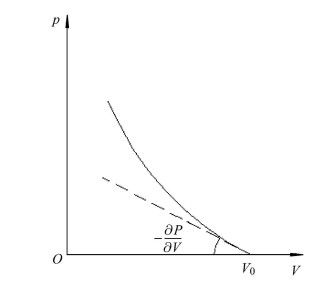图 1 波速$C$取决于$P$-$\!$-$V$曲线的局部斜率($- \partial P / \partial V)$ Figure 1 The wave velocity $C$ depends on the local slope of $P$-$\!$-$V$ curve ($- \partial P / \partial V)$

2 脉搏波的反演

“切脉象"主要是用手指感受脉搏的压力波.当采用$n$个压力计在$n$个物质坐标$X_{i}$($i=1, 2, \cdots, n$)处测知$n$个压力波剖面$P(X_{i}, t)$时，其一阶偏导数$\partial P/\partial t$$\partial P/\partial X可用数值微分计算确定, 再由动量守恒方程(式(1))即可求得\partial v / \partial t.如果利用通常实验条件下的初始条件：t=0$$v=0$，不难对$\partial v / \partial t$通过对时间的积分来求得$v(X_{i}, t)$.

“寸口三部九候诊法"中的所谓“九候"，乃指寸、关、尺三部“每部各以轻、中、重指力按脉，分浮、中、沉" (《难经$\cdot$十八难》).寸关尺三部在不同压力的组合下, 可以测得不同的脉搏波信息.一方面，中医切脉时通过不断调节3个指头的压力, 可以达到三部最满意的指感；另一方面，对比轻、中、重按所获得的不同脉搏波信息，中医可以获得更多诊察信息.从脉搏波反演系统本构关系的角度看，不同指压下获得的脉搏波会具有不同压力幅值和不同波形信息，将会有利于定量识别本构关系可能存在的非线性程度和黏滞性大小，这是脉搏波反演下一步需要进一步探索研究的.这种操作是传统的拉氏反分析原本所没有的，因此也值得拉氏反分析应用于其他研究领域时加以吸取和发展.

“三部九候诊法"还有另一理解，即在全身遍诊法中，乃指“于头部、上肢、下肢三部诊脉，每部各有上、中、下动脉，不同部的脉出现独大、独小、独迟、独数，即表示该经的脏气有寒热虚实之变化". 图 2图 3是作者王晖在进行“三部九候"的全身遍诊的实例.医生在进行全身遍诊法时，两只手对头部、上肢、下肢三部任选两部进行脉诊，今后用科学的仪器同时测得三部信息，必将提供更多有效信息.从连续介质力学波动理论的角度看，这将有利于识别脉搏波的衰减、弥散和耗散特征，进而加深对系统本构关系类别的定量识别.这方面目前还缺乏定量研究，正是需要进一步探索的新领域.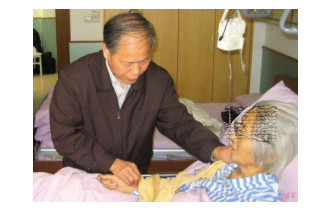图 2 对上肢和下肢两部同时进行脉诊 Figure 2 Pulse-taking on the upper limb and lower limb simultaneously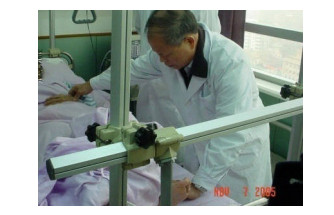图 3 对头部和上肢两部同时进行脉诊 Figure 3 Pulse-taking on the head and upper limb simultaneously
3 由脉搏波本构关系联想中医的“体质观"

3.1 非线性效应

(1)与线性波速为恒值的规律不同，脉搏波波速随脉搏波压力高低而变化，不是恒值.

(2)每一次的脉搏收缩与舒张会有额外的能量耗散.表现在随脉搏波的传播，波形会发生波幅变化和能量耗散.

(3)与线性波不同，非线性脉搏波的压力波$P(X, t)$，质点速度波$v(X, t)$，比容波$V(X, t)$和内能波$E(X, t)$之间不存在正比的线性关系.

3.1.1 递增强化非线性效应

(1)按照式(5(b))脉搏波波速取决于$P-V$曲线的局部斜率($C = V_0 \sqrt { - \partial P / \partial V}$)，因而“递增强化"非线性特性就意味着，压力高的扰动之传播速度大于压力低的.换言之，在脉搏的收缩过程中，后施加的压力高的扰动将追赶先施加的压力低的扰动，最后形成具有陡峭波阵面的所谓“冲击波(shock wave)"，具有陡峭的波前沿(升支).

(2)然而，在脉搏舒张过程中则由于较高压力扰动先卸压，比后卸压的较低压力扰动传播得快，就不会形成冲击波.而形成带有震荡弥散性质的连续波.于是，在脉搏一缩一张时，脉搏波的上升部分和下降部分分别呈现不同传播特性.

(3)由此，每一次的脉搏收缩与舒张会有额外的能量耗散.脉搏波在传播过程中会发生波幅衰减和能量耗散.

3.1.2 递减强化非线性效应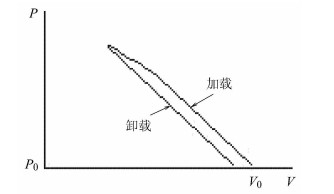图 4 递减强化$P$-$\!$-$V$曲线 Figure 4 The decreasingly-hardening $P$-$\!$-$V$ curve

(1)根据式(5(b))，即$C = V_0 \sqrt { - \partial P / \partial V}$，“递减强化"非线性特性意味着，在脉搏的收缩过程中，后施加的压力较高的扰动之传播将慢于先施加的压力较低的扰动传播，因而随着脉搏波的传播，波阵面与波尾的距离愈来愈大，形成波形弥散的连续波.

(2)在脉搏舒张过程中，由于卸载扰动的传播快于较高压力加载扰动的传播，将出现卸载波追赶加载波、并相互作用的情况，使问题大为复杂.

(3)而且，由于脉搏的收缩和舒张遵循不同的$P-V$关系，每一次的脉搏收缩与舒张会有额外的能量耗散(对应于图 4中加载曲线与卸载曲线所包围的面积).脉搏波在传播过程中会发生能量耗散和由于卸载波追赶加载波导致的波幅衰减.

3.2 黏性效应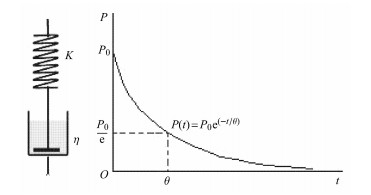图 5 Maxwell黏弹性模型(左)及其松弛特性(右) Figure 5 Maxwell visco-elastic model (left) and its relaxation character (right)
 $\dfrac 1{V_0}\dfrac{\partial V}{\partial t}+\dfrac 1K \dfrac{\partial P}{\partial t}+\dfrac P \eta=0$ (6)

 $P(t)=P_0\exp \Big ( -\dfrac{Kt}{\eta} \Big )=P_0 \exp \Big (-\dfrac t{\theta} \Big )$ (7)

(1)波速由串联的弹簧组件特性决定，即有

$C_0=\sqrt{K/\rho_0}={\rm const.}$，而黏性系数$\eta$的影响表现在：黏弹性波的强度(最大波幅)将从初始值$P_{0}$按如下指数规律衰减[9, 33]

 $P=P_0\exp \Big ( -\dfrac{\rho_0C_0}{2\eta} X \Big ) =P_0 \exp ( -\alpha X )$ (8a)

$\alpha$称为衰减因子

 $\alpha = \dfrac{\rho _0 C_0 }{2\eta }$ (8b)

(2)脉搏舒张时，脉搏压力开始下降了，但由于黏性效应，比容并不立即随之下降，会延续一个短时间的继续增加，然后才下降.

(3)因此在$P$-$\!$-$V$图上，与脉搏收缩与舒张所对应的加载曲线与卸载曲线形成滞回曲线，两者所包围的面积代表每一次脉搏收缩与舒张所损耗的能量.

(4)黏弹性脉搏波的质点速度波$v (X, t)$、比容波$V (X, t)$和内能波$E (X, t)$等，也与压力波$P (X, t)$一样，随传播距离以指数规律衰减，但当计及非线性效应时四种形式波之间不再有线性正比关系[9, 33].

4 结语和展望

(1)脉搏波是压力波$P (X, t)$，质点速度波$v (X, t)$、比容波$V (X, t)$和内能波$E (X$, $t)$这些不同形式波动的总成.只凭传感器测得的单个压力波$P (X, t)$或质点速度波$v (X, t)$等来模拟中医的脉诊是不完整的.

(2)脉搏现象包含着血液的流动和在血液中传播的携带能量的脉搏波.前者是人眼易见的实体血液的“物质流"，而后者是人眼不易见、以波的形式传播的“能量流"，分别对应地可理解为中医的“血"和“气".血是气传播的物质载体(媒介)，而气是以波形式传播的推动血运行的能量，两者既密切相关不可分，又互有区别不可混.

(3)脉搏波传播速度$C$ (10$^{0}$$\sim$10$^{1}$ m/s量级)比血液质点运动速度$v$(10$^{ -1}$ m/s量级)快1$\sim$2个量级.远早于血液流动的到达，脉搏波已以更快的速度把能量和血液运动状态变化传递给了人体各部分.

(4)脉搏波的传播特性并不仅仅由心脏和动脉血管来决定，而由反映生命体整体系统的本构方程决定.生命体的任何局部出现病态，反过来就会影响脉搏波.脉搏波灵敏地反映着生命实体(广义之血)和生命能量(广义之气)不断运行变化着的生命状态.

(5)把力学反演方法推广到脉搏波，可由实测的一系列某形式脉搏波信息(例如压力波$P (X, t)$)，通过守恒方程，定量地反演其它形式脉搏波，以及脉搏波系统的本构方程.

(6)脉搏波系统本构关系(constitutive relation)就其重要性和地位而言，与中医的体质(body constitution)相当.如何把通过反演获得的不同类型脉搏波系统本构方程与中医的不同类型体质，建立内在联系，是值得展望的重要研究方向.本构方程的非线性效应和黏性效应在反映脉搏波传播特性和体质类型上可能是不可忽略的因素.

Wang Lili*1), Wang Hui